### Abstract:

In this paper we prove the Upper Bound Conjecture (UBC) for some classes of (simplicial) homology manifolds: we show that the UBC holds for all odd-dimensional homology manifolds and for all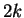-dimensional homology manifolds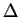such that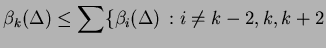and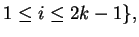where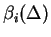are reduced Betti numbers of. (This condition is satisfied by-dimensional homology manifolds with Euler c haracteristic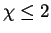when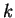is even or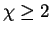whenis odd, and for those having vanishing middle homology.)

We prove an analog of the UBC for all other even-dimensional homology manifolds.

Kühnel conjectured that for every-dimensional combinatorial manifold with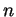vertices,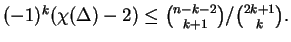We prove this conjecture for all-dimensional homology manifolds withvertices, where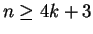or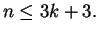We also obtain upper bounds on the (weighted) sum of the Betti numbers of odd-dimensional homology manifolds.